# Coding-Decoding

## Introduction

Coding-decoding in aptitude exams is a significant part of logical reasoning. This can be either for words, numbers or patterns that need to be encrypted or decrypted.

• The coding process is used to encrypt words or numbers in the code or pattern based on a specific set of rules.
• The process of decoding is decrypting the patterns into the original words or numbers from the given codes.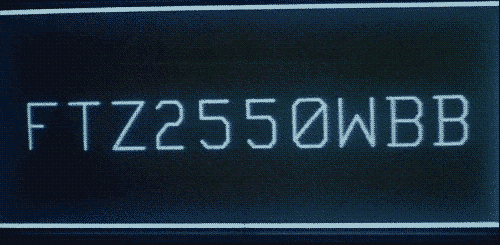Source: gifer.com

### Letter coding

Such questions have alphabets of a word replaced by other alphabets according to some particular rule to form code or following some specific pattern.

Example: In a certain code, HELLO is written as IGOPT.  What would SCHOOL be written as using the same code?

### Number coding

Such questions have alphabets of a word replaced by numbers following some specific pattern.

Example: In a certain code, HELLO is written as 52.  What would FIRE be written as using the same code?

## Sample Questions

Q1. Using some certain coding, 15789 is written as XTZAL and 2346 is written as NPSU. How will 23549 be written in that code?

Answer: 23549 would be written as NPTSL using coding because the numbers are coded as alphabets like 5 as T, 2 as N, 4 as S and 9 as L in given data.

Q2. Coding the word DELHI using a particular pattern, the word is written as EDMGJ. How would PERSON be written in the same code?

Answer: Every alphabet of DELHI is increased by one and the result of coding it is a word with the next letter of respective alphabets. So, PERSON would be written as QFSTPM.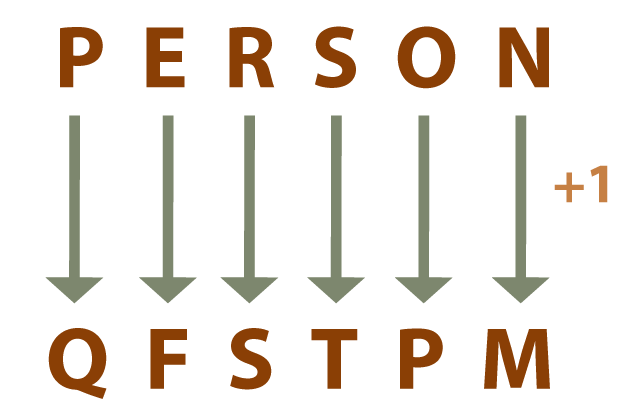Q3. Coding the word MOON using a particular pattern, the word is written as NQRR. How would FOREST be written in the same code?

Answer: FOREST would be written as GQUIXZ on coding it because the first alphabet in MOON is increased by 1, the second alphabet by 2, the third by 3 and so on.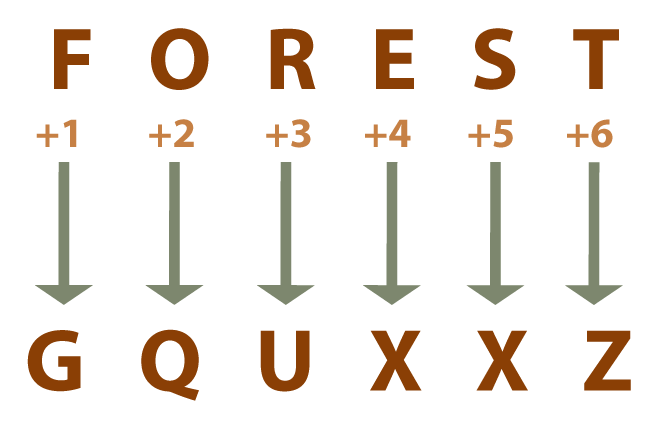Q4. In a certain language, BIRTHDAY is written as TRIBYADH. How is MOUNTAIN written in this code?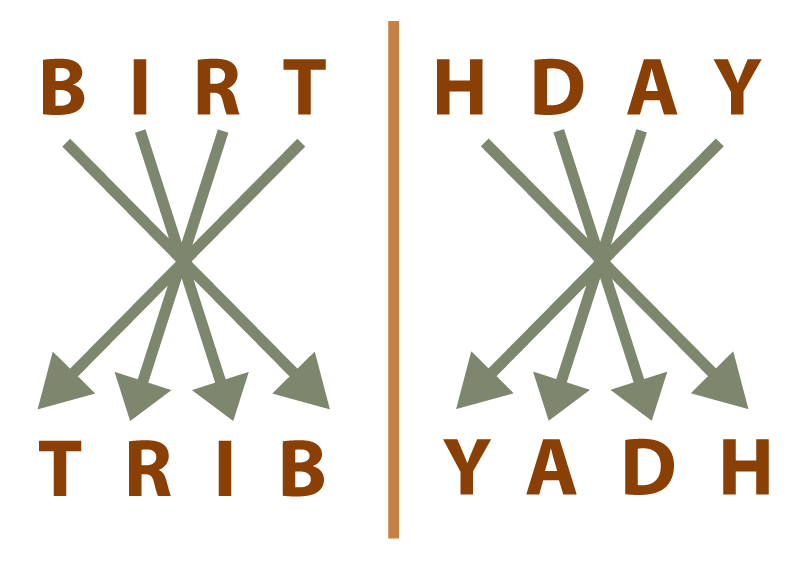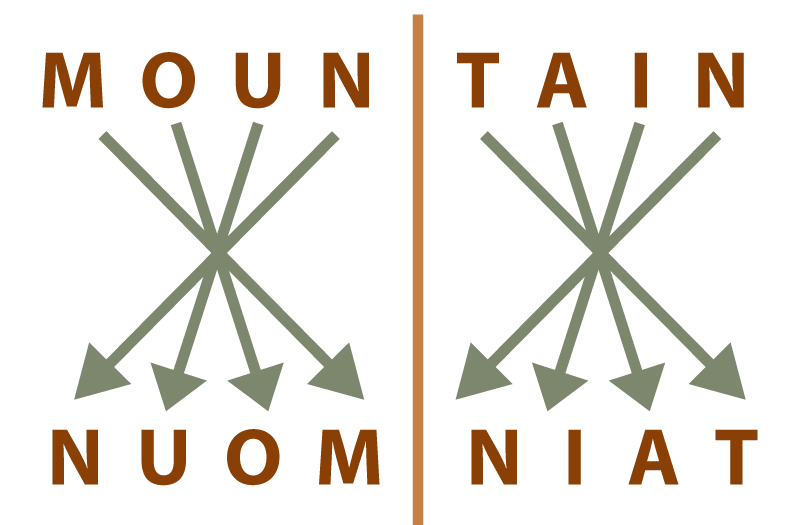Q5. In a certain code, NUMBER is written as NVOSFC. What would be MOBILE coded as?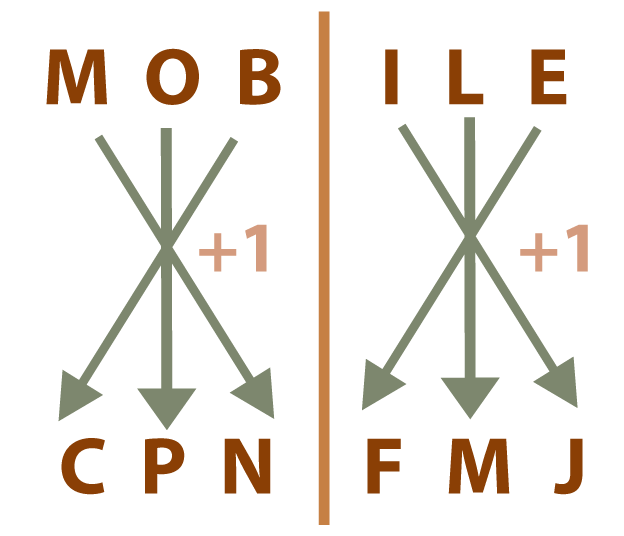Q6. In a certain code, NEWJERSEY is written as 124. What would NUMBER be written as on coding?

Answer: In NEWJERSEY, 14+5+23+10+5+18+19+5+25=124. Using a similar pattern while coding NUMBER, 14+21+13+2+5+18=73. So, NUMBER would be 73.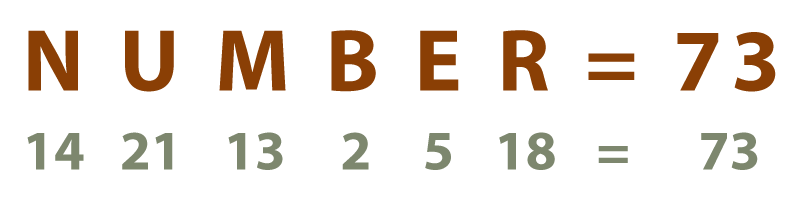Q7. In a certain code, STARE is written as 27. What would be the code for ACTIVE using the same pattern?

S=19, 1+9=10

T=20, 2+0=2

A=1

R=18, 1+8=9

E=5

Therefore, ACTIVE would be 24.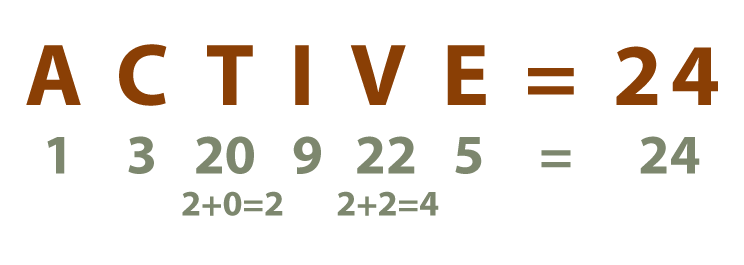Q8. If BREAD is coded as CQFZE in a certain language, which word would be written as TVJOF?

Answer: Every alphabet at odd places in the word is one step behind and every alphabet at even places is one step ahead of the corresponding alphabets in the code.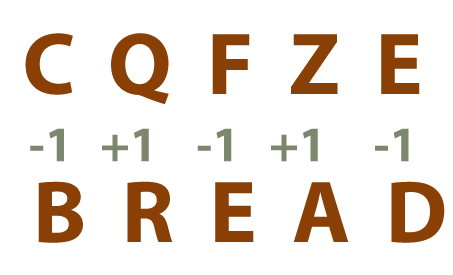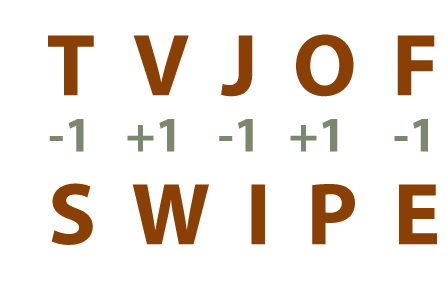Q9. If 256 means “you are good”, 637 means “you are bad” and 358 means “good and bad” in a certain code, then which number represents “and” in that code?

Options: 3, 10, 8, 4

Answer: The common digit in the first and third statements is 5 and the common word in both these statements is “good”. This implies that 5 means “good”.  The common digit in the second and third statements is 3 and the common word is “bad”, meaning that 3 is for “bad”. Therefore, 8 means “and” in the third statement.

Q10. If ‘nee tim see’ means ‘how are you’ in a certain code, and ‘ble nee see’ means where are you, then what is the code for where?

Answer: The common code in the first and second sentences is ‘nee’ and ‘see’ and the common words are ‘are’ and ‘you’. So in the second sentence, the remaining code is ‘ble’ which is the code for ‘where’.

Ques 1. What is the difference between coding and decoding?

Ans. Coding means encrypting words or numbers in a code while decoding refers to decrypting the patterns into the original words or the original form of the code.

Ques 2. What are the main kinds of coding questions?

Ans. Coding-decoding questions usually have the following types: Letter coding, number coding, substitution coding and mixed coding.

Ques 3. What is substitution coding?

Ans. Substitution coding is the kind of coding where particular words are assigned to words or phrases. The given phrase could have a substituted name that needs to be decoded.

## Key takeaways

In this article, we learned about the concepts of coding, decoding and their types along with sample questions of all kinds for practice.

You can go to CodeStudio to try and solve more problems for practice. Send this blog to your friends if you found it helpful! Until then, All the best for your future endeavours, and Keep Coding.